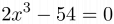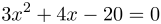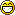Root Solver

Write a program that solves for the root of a univariate polynomial with a maximum degree of 100.

Input Format

Line 1 of the input contains an integer T (1 ≤ T ≤ 101), indicating the number of terms in the polynomial.
The next T lines each contain a real number coefficient and a whole number exponent from 0 to 100 inclusive.
Terms will always be collected (i.e. exponents will not be repeated) and arranged in descending powers.

Output Format

In order from lowest value to highest value, every real solution for when the polynomial is equal to zero.
Your answer must be accurate within ±10-5 in absolute and relative error.
If there are no real solutions to the equation, output "NO REAL ROOTS".

Note: Coefficients and answers can have up to 18 digits, and will fit inside a long double.

2
2 3
-54 0

3

Explanation

This asks for the solution(s) of x for the equation$\displaystyle 2x^3-54=0$.

3
3 2
4 1
-20 0

-3.33333
2

Explanation

This asks for the solution(s) of x for the equation$\displaystyle 3x^2+4x-20=0$.

Point Value: 30 (partial)
Time Limit: 2.00s
Memory Limit: 16M
Author: Alex

Languages Allowed:
C++03, PAS, C, HASK, ASM, RUBY, PYTH2, JAVA, PHP, SCM, CAML, PERL, C#, C++11, PYTH3

• (1/0)
10 new cases are added. You now need up to 18 digits of precision. Please use a long double (C++) or extended (Pascal) to hold the coefficients and answers. Keep in mind that even these datatypes may still not be large enough to have the operational precision, depending on which algorithms you use. Jacob, I've fulfilled your requests for worst cases. As a result your own solution gets 69/100. You've brought this upon yourself.• (1/0)
Thanks for the update! These cases are indeed pretty rough =P
But good, I didn't expect my program to actually work right away.

• (1/0)
I changed case 15 a bit because I suspected that Mathematica may have poorly rounded complex roots. You deserved that perfect, so happy birthday.

• (0/0)
Ah, that's nice! I had given up on this one due to being completely unable to calculate these values with enough precision (or so I thought).

• (2/5)
Alex super hacker OP

• (0/0)
I'm confused - the author's solution only works for certain, small kinds of cases, and is certainly not a legitimate solution to the general problem being asked.

If this is really what's intended, then the problem statement should reflect it, and the point value should be reduced. Otherwise, larger cases should be added.

• (2/1)
I should have been more detailed about coefficients, but I thought it was fair to make the assumption that the coefficients in the input will fit inside a double. Assuming this, it's possible to mathematically prove that the root(s) will only lie in a certain range, and then make estimations about where to apply the bisection method. As a result, the time limit had been set liberally. I apologize if I've caused anyone trouble, so I'll specify now that the coefficients will only have around 10 significant digits. I dare not admit to any officialness in anything I submit right now and I assure you that it's not my final attempt. I'm just using this judge as an ongoing way to test different methods and find solutions myself. You can blame Daniel for the value, but for now, please ignore my submissions.

• (0/0)
When I first gave a point value, I assumed that the test data would be more rigorous and the roots would be within a broader range. Due to a recent turn of events, the point value of the problem has been reduced to 15.

• (1/0)
As the polynomial's degree increases, the possibility for larger roots decrease. The larger test data was generated 100% randomly, although the smaller ones could have been more cleverly crafted. Like I said, ignore my submissions for now as I'm trying to explore different ways to solve it myself. Currently, solutions have been rejudged.

• (1/0)
Okay, I see. So, this is where it's important to use not only random cases, but worst-case ones against certain algorithms (for example, it's not hard to develop cases with roots with extreme magnitudes, multiple roots extremely close together, points where the polynomial touches the x-axis but doesn't pass through it, etc.). If such cases could be included, I think 25 points would be an appropriate value for this problem, as it's quite tricky to code.